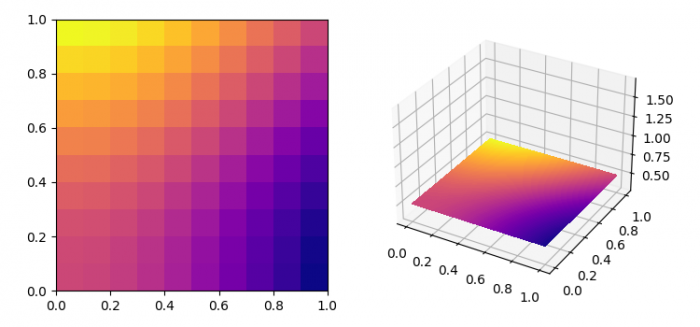# Plotting an imshow() image in 3d in Matplotlib

To plot an imshow() image in 3D in Matplotlib, we can take the following steps −

• Create xx and yy data points using numpy.

• Get the data (2D) using X, Y and Z.

• Create a new figure or activate an existing figure using figure() method.

• Add an 'ax1' to the figure as part of a subplot arrangement.

• Display the data as an image, i.e., on a 2D regular raster with data.

• Add an 'ax2' to the figure as part of a subplot arrangement.

• Create and store a set of contour lines or filled regions.

• To display the figure, use show() method.

## Example

import matplotlib.pyplot as plt
import numpy as np
from matplotlib import cm

plt.rcParams["figure.figsize"] = [7.50, 3.50]
plt.rcParams["figure.autolayout"] = True

xx, yy = np.meshgrid(np.linspace(0, 1, 10), np.linspace(0, 1, 10))

X = xx
Y = yy
Z = 10 * np.ones(X.shape)

data = np.cos(xx) * np.cos(xx) + np.sin(yy) * np.sin(yy)
fig = plt.figure()

plt.show()